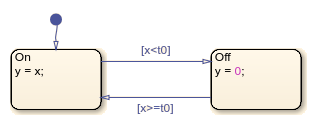# getReferences

Identify references to symbol name

Since R2023a

## Syntax

``references = getReferences(symbol)``

## Description

example

````references = getReferences(symbol)` returns an array of `Stateflow.SymbolReference` objects that indicate where the chart refers to a symbol name. For example, a `Stateflow.SymbolReference` object can correspond to a state or transition action that includes the symbol name. Symbols include `Stateflow.Data`, `Stateflow.Event`, `Stateflow.Message`, and other Stateflow® objects that have a `Name` property.```

## Examples

collapse all

Rename and update the references to the chart output `y`.Open the model and access the `Stateflow.Data` object for the chart output `y`.

```open_system("sfRectifyAPIExample") data = find(sfroot,"-isa","Stateflow.Data",Scope="Output"); data.Name```
```ans = 'y' ```

Find the locations where the chart refers to the chart output.

`references = getReferences(data)`
```references=2×1 object 2x1 SymbolReference array with properties: Position WhereUsed ```

Display the names and entry actions of the states that refer to the chart output.

```whereUsed = [references.WhereUsed]; classes = arrayfun(@class,whereUsed,UniformOutput=false); idx = (classes=="Stateflow.State"); states = whereUsed(idx); get(states,{"Name" "EntryAction"})```
```ans = 2x2 cell {'On' } {'y = x;'} {'Off'} {'y = 0;'} ```

Change the `Name` property of the chart output to `"z"` and update the references to the output in the chart.

```renameReferences(data,"z") data.Name```
```ans = 'z' ```

Display the names and modified entry actions of the states that refer to the chart ouput.

`get(states,{"Name" "EntryAction"})`
```ans = 2x2 cell {'On' } {'z = x;'} {'Off'} {'z = 0;'} ```

collapse all

## Version History

Introduced in R2023a